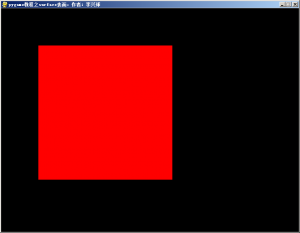pygame教程之关于surface

>>> import pygame
>>> pygame.init()
(6, 0)
>>> width,height = 800,600
>>> screen = pygame.display.set_mode((width,height))
>>> type(screen)
<class 'pygame.Surface'>
>>>

>>> square = pygame.Surface((360,360))
>>> square.fill((255,0,0))
<rect(0, 0, 360, 360)>
>>> screen.blit(square,(100,100))
<rect(100, 100, 360, 360)>
>>> pygame.display.update()
>>>

"""
pygame教程之surface表面

"""

import pygame                      # 导入pygame模块

pygame.init()                      # pygame初始化

width,height = 800,600             # 定义全局变量

screen = pygame.display.set_mode((width,height)) # 新建屏幕，它是一个surface

pygame.display.set_caption("pygame教程之surface表面，作者：李兴球")

square = pygame.Surface((360,360)) # 新建square，它是一个surface

square.fill((255,0,0))             # 填充square为红色

screen.blit(square,(100,100))      # 把square贴在screen上

pygame.display.update()            # 刷新合成后的屏幕显示

#pygame.quit()             # 开了这个会一闪而过"""

"""

import pygame                      # 导入pygame模块

pygame.init()                      # pygame初始化

width,height = 800,600             # 定义全局变量

screen = pygame.display.set_mode((width,height)) # 新建屏幕，它是一个surface

pygame.display.set_caption("pygame教程之surface表面，作者：李兴球")

square = pygame.Surface((36,36)) # 新建square，它是一个surface

square.fill((255,0,0))             # 填充square为红色

x , y = 100,100                    # 代表矩形的渲染坐标
dx = 1                             # 代表矩形每次移动的水平距离

for n in range(500):
screen.fill((0,0,0))           # 填充屏幕为黑色
screen.blit(square,(x,y))      # 把square贴在screen上
x = x + dx                     # 水平坐标增加dx
pygame.display.update()        # 刷新合成后的屏幕显示

#pygame.quit()             # 开了这个会窗口会关闭